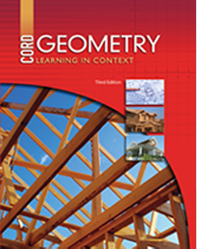# Geometry - Third Edition

## Chapter 8: Perimeter and Area

Some links are repeated for use with more than one lesson.

Please report broken links to Kathy Kral

### These sites apply to the entire chapter.

http://www.freemathhelp.com/Lessons/PreAlgebra_Perimeter_and_Area_BB.htm
This site contains a video tutorial with notes on perimeter and area.

http://staff.argyll.epsb.ca/jreed/math9/strand3/3108.htm
This page summarizes and illustrates the perimeter and area formulas of the basic geometric figures, along with a word search applets for vocabulary terms. (Requires Java.)

http://www.regentsprep.org/regents/math/algebra/AS1/indexAS1.htm
This page includes several links to discussion and practice of areas and perimeters for the polygons covered in this chapter.

### 8.1 Rectangles and Squares

http://www.syvum.com/cgi/online/serve.cgi/squizzes/geometry/square_rectangle_area_1.html
This page gives the formulas for finding perimeter and area of rectangles and squares.

http://www.cimt.plymouth.ac.uk/projects/mepres/book7/bk7i9/bk7_9i3.htm
This page explains and providers practice for finding the perimeter and area of rectangles and squares.

### 8.2 Parallelograms and Triangles

http://www.cut-the-knot.org/Curriculum/Geometry/AreaOfParallelogram.shtml
This site shows how to find the area of a parallelogram.

### 8.3 Trapezoids

http://staff.argyll.epsb.ca/jreed/math9/strand3/trapezoid_area_per.htm
This page interactively demonstrates the formulas for finding the perimeter and area of trapezoids.

http://www.algebralab.org/Word/Word.aspx?file=Geometry_AreaPerimeterTrapezoids.xml
This page contains practice problems on finding the perimeter and area of trapezoids.

### 8.4 Regular Polygons

http://www.algebralab.org/lessons/lesson.aspx?file=Geometry_AreaPerimeterRegularPolygons.xml
This site explains how to find the perimeter and area of regular polygons.

http://www.efunda.com/math/areas/RegularPolygonGen.cfm
This page discusses the perimeters and area of regular polygons.

### 8.5 Circles

This page gives the formulas for finding perimeter and area of a circle.

http://www.analyzemath.com/Geometry_calculators/area_perimeter_circle.html
This page contains a calculator that finds the perimeter and area of a circle by stating the radius.

### 8.6 Areas of Similar Figures

http://www.explorelearning.com/index.cfm?method=cResource.dspDetail&ResourceID=189
This page contains a gizmo about perimeter and area of similar figures.